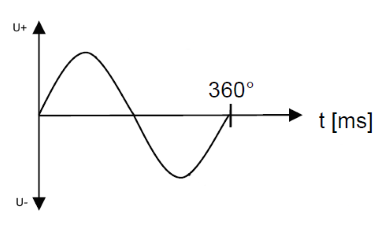Calculate frequency and period

Online calculators and formulas for calculating the frequency and period of AC voltages

Calculate frequency or period

On this page you can calculate the duration of the period for a certain frequency, or the frequency for a period.

Period and frequency calculator

 What should be calculated? Period Frequency Input Frequency Hz kHz MHz GHz Period pSek nSek µSek mSek Sek Decimal places 0 1 2 3 4 6 8 10 Result Period Frequency

Tip: A calculator for calculating frequency and wavelengths can be found here

Formula for frequency and period timeThe frequency is the number of periods per second. The formula below applies to the calculation of the frequency:

$$\displaystyle f= \frac{1}{T}$$

The duration of a period is calculated using the following formula:

$$\displaystyle T= \frac{1}{f}$$

Legend

 $$\displaystyle f$$ Frequency in Hz $$\displaystyle T$$ Duration of a period in seconds